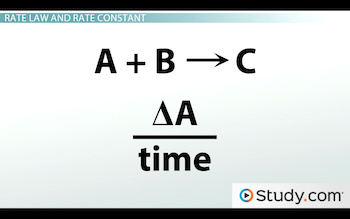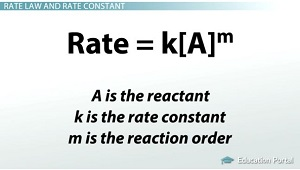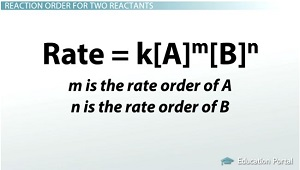# Rate Constant and Rate Laws

An error occurred trying to load this video.

Try refreshing the page, or contact customer support.

Coming up next: Rate of a Chemical Reaction: Effect of Temperature

### You're on a roll. Keep up the good work!

Replay
Your next lesson will play in 10 seconds
• 0:06 Rate Law and Rate Constant
• 1:59 Reaction Order for One…
• 4:15 Rate Law and Rate…
• 5:45 Lesson Summary
Save Save

Want to watch this again later?

Timeline
Autoplay
Autoplay
Speed Speed

#### Recommended Lessons and Courses for You

Lesson Transcript
Instructor: Amy Meyers

Amy holds a Master of Science. She has taught science at the high school and college levels.

Learn what the rate law is and how the rate constant relates to it. Learn what reaction order is and how to determine reaction order when given experimental data containing concentration and reaction rate.

## Rate Law and Rate Constant

Pretend you are out camping. You are cold and wet, and you want to build a big fire quickly to dry out and warm up. You get a little flame going, but how best do you get a big fire quickly? Do you add oxygen to it? More wood? Lighter fluid? What reactant can you add to make the rate of the reaction happen quicker?

The question you are really asking is, 'What is the reaction mechanism?' How exactly does the reaction that makes fire work? What reactants must be brought together in what concentrations to make the final product? When studying chemical reactions, the rate law allows scientists to determine factors like these.

You have learned that the rate of a reaction is affected by the concentration of the reactants. You know that rate is change over a period of time. For example, a reaction of A + B --> C has a rate of that below:The delta symbol above means 'change in.'

It should come as no surprise after studying chemistry for a while that there is a law for rate. It is called the rate law. The rate law is the relationship between the rate of a reaction and the concentration of the reactants. The equation for the rate law is:

Rate = k[A]^m

A is the reactant
k is a constant called the rate constant
m is the reaction order

If m = 0, then the reaction is zero order, and the rate is independent of the concentration of A.
If m = 1, then the reaction is first order, and the rate is directly proportional to the concentration of A.
If m = 2, then the reaction is second order, and the rate is proportional to the square of the concentration of A.## Reaction Order for One Reactant

Now you might be wondering what determines the order of a reaction. The order of a reaction is determined by data gathered during experimentation. There is no way to determine the order by just looking at a reaction equation. It must be determined by experiment.

Once you have the experimental data, you can determine the k by comparing the concentration of A and the rate of the reaction. If the concentration of A increases but the rate remains the same, it is a zero order reaction because the reaction rate is independent of the concentration of A. If the concentration of A and the rate increase at the same rate, then the reaction order is one because they are directly proportional. If the rate quadruples for every doubling of A, then the reaction order is two because the rate is proportional to the square of the concentration.

Let me show you a table to try to make this clearer. The table below shows a reaction order of zero. Notice how the rate of the reaction doesn't change even when the concentration increases.

Concentration of A Rate of Reaction
0.1 0.15
0.2 0.15
0.4 0.15

The next table shows a reaction order of one. Notice how the rate of the reaction increases at the same rate as the concentration increases.

Concentration of A Rate of Reaction
0.1 0.15
0.2 0.30
0.4 0.60

The next table below shows a reaction order of two. The rate of the reaction quadruples for every doubling of the concentration.

Concentration of A Rate of Reaction
0.1 0.15
0.2 0.60
0.4 2.40

## Reaction Order for Two Reactants

Reaction order can also be determined for reactions with two reactants. For the reaction A + B --> C + D, the rate equation is:

Rate = k[A]^m[B]^n

…where m is the rate order of A and n is the rate order of B. The rate order of the overall reaction is m + n.Let's try a problem that deals with the rate law.

To unlock this lesson you must be a Study.com Member.

### Register to view this lesson

Are you a student or a teacher?

#### See for yourself why 30 million people use Study.com

##### Become a Study.com member and start learning now.
Back
What teachers are saying about Study.com

### Earning College Credit

Did you know… We have over 200 college courses that prepare you to earn credit by exam that is accepted by over 1,500 colleges and universities. You can test out of the first two years of college and save thousands off your degree. Anyone can earn credit-by-exam regardless of age or education level.